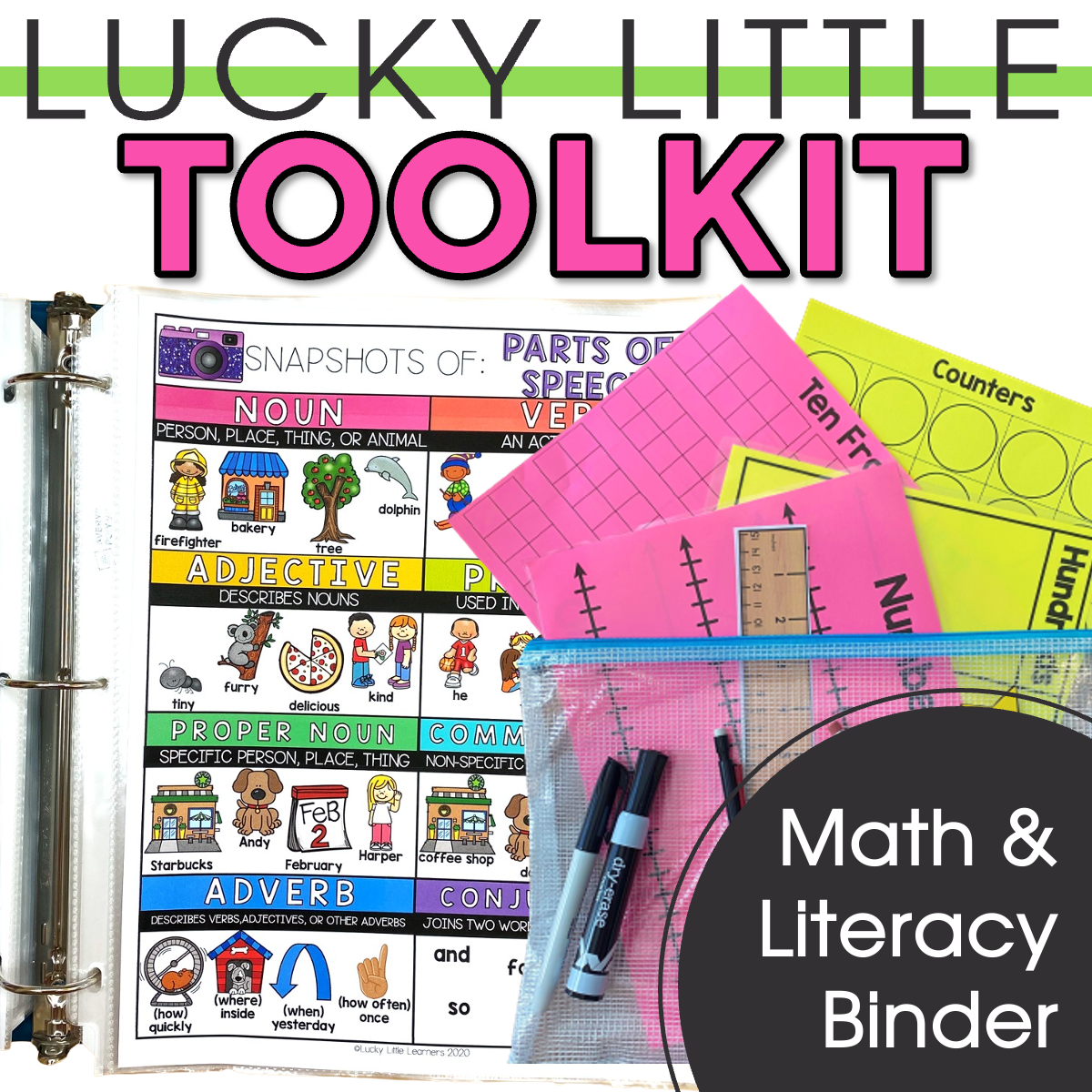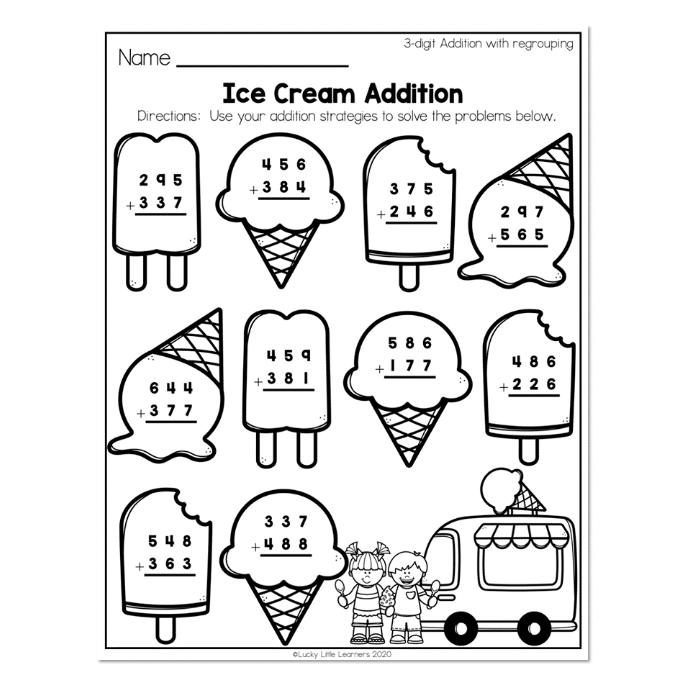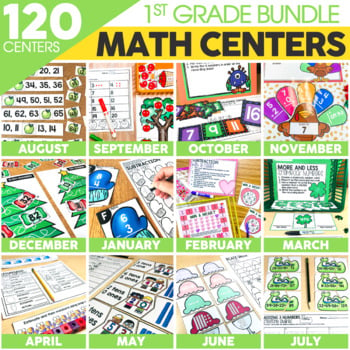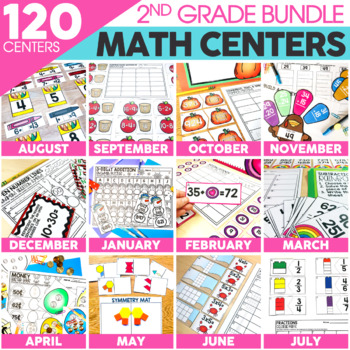Home » Blog » 2nd Grade Skills » Operations » Teaching Addition & Subtraction Within 1,000

# Teaching Addition & Subtraction Within 1,000

Written by: Katie Palmer

Addition is almost always taught before subtraction. Once students are confident with addition strategies, it is easier to teach subtraction! The strategies and resources below are sure to get your students adding in no time!

Before you get into 2 or 3-digit addition, make sure students have mastered their basic addition facts. This post is your first stop: 14 Strategies for Teaching Addition.

Second stop: build strong basic math foundations with the strategies in the following post:

### 2- Base Ten Blocks & Other Manipulatives

Using hands-on manipulatives is a GREAT way for students to see how addition works! By adding more blocks they can actually see the answer being made! This can also be a great time to show how tens and hundreds are made! (By trading ten 1s for a tens block, etc..)

Don’t have base ten blocks? No problem! This website: Larson Mathematical Practices has LOADS of pdf printable math manipulatives, including Base Ten Blocks.

If you would prefer printable manipulatives in a more kid friendly format, check out the Lucky Little Toolkit!

Read more about the Lucky Little Toolkit here: Lucky Little Toolkit (Lifesaver!)### 3- Partial Sums Method

This is not your mom’s way of adding! The partial sums method is a way to add mentally and quickly! Students who use this method sometimes write down their partial sums when they are still mastering adding bigger numbers. How does this method work? Follow the steps below.

Let’s solve this problem using the partial sums method:

42 + 35

1. Add the tens first: 40 + 30 = 70 (Some students will want to write that number model so they don’t forget their tens answer.)
2. Add thee ones place: 2 + 5 = 7
3. Put them together: 70 + 7 = 77

This is a great strategy! Students will get very comfortable adding big numbers this way! When your class is ready, move on to adding numbers in the hundreds as well!

This video is a great visual example of the partial sums method in action! (And would be great to show parents too!)

Check out this post: Activities for Practicing 2-Digit Addition for more ideas!

### 4- Videos that Teach Addition

We all know students like short, engaging learning videos! Head to this post: Kid Friendly Videos that Teach Addition for some great options!

## 5 – Addition Strategy Anchor Charts

Looking for more ways to help students recall addition strategies? Our Lucky to Learn Math Curriculum has illustrated anchor charts for 2nd grade addition and subtraction. These anchor charts are great o print and post around the classroom, use for whole group or small group instruction, or print for each student to keep in the math notebook for reference.

Anchor Charts for 2 and 3 Digit Addition

## Subtraction Strategies

As with addition, there are a few helpful ways to teach subtraction. Try using one (or all) of the strategies listed below to get your students subtracting seamlessly!

### 1- Drawing Base Ten Blocks

Yep, we are mentioning base ten blocks again! But this time, just using base ten shorthand and drawing the blocks! Here is the why and the process:

### 2- Partial Sums Subtraction

We can also use the partial sums method when subtracting, but this method is best used when there is NO regrouping.

Let’s solve this problem using the subtraction partial sums method:

584 – 351

2. Move on to the tens: 80 – 50 = 30
3. Now the. ones: 4 – 1 = 3
4. Now add up all three of your partial sums: 200 + 30 + 3 =233

### 3- Videos that Teach Subtraction

Like I mentioned above, students love quick learning videos! Check out this post for kid friendly options: Videos that Teach Subtraction

Ready to have students practice these new strategies?! Check out these awesome resources!

### 1- Two Digit & Three Digit Addition & Subtraction Worksheets

Straight up practice is the number one way to improve student math confidence! Using these 2-Digit & 3-Digit Addition & Subtraction worksheets is a great place to start as it includes 140 digital & printable practice sheets! Learn more about how to use our worksheets to build up to three digit subtraction with regrouping.

Take a closer look at this resource:### 2- 2nd Grade Math Centers

Using centers is always a GREAT option. Students feel like they are playing games, you know they are learning and practicing math strategies! This resource includes over 1,200 pages of center materials!

Read EVERYTHING Math centers here: Math Centers### 3- Toothy

There is NO way we could leave Toothy off this list! Toothy is a FAVORITE in the classroom. An added bonus to this game is once students know how to play, they can use it to review ANY subject/skill. (The teacher just needs to come up with review questions!)

Read all things Toothy here: Toothy

## 4 – Subtraction Strategy Anchor Charts

Looking for more ways to help students recall subtraction strategies? Our Lucky to Learn Math Curriculum has illustrated anchor charts for 2nd grade addition and subtraction. These anchor charts are great o print and post around the classroom, use for whole group or small group instruction, or print for each student to keep in the math notebook for reference.

Anchor Charts for Basic Subtraction

Anchor Charts for 2 and 3 Digit Subtraction